### Notes of Artificial Intelligence [CT 653]

#### Machine Learning

Concept of Machine Learning

- Learning is the process of acquiring new knowledge or modifying existing knowledge to adapt to new situations.
- Learning involves 3 factors as:
a) Changes
- Learner changes
- Problem is to determining the change and to represent them in efficient way
b) Generalization
- Performance improves on all similar tasks
c) Improvement

Learning by Analogy, Inductive learning and Explanation based Learning

Rote Learning:

- Technique in which the system stress all the information computed before.
- When need, those information are retrieved rather than recompilation.
- Useful if time to retrieve is less that time to compute
- Eg: checker playing program of Samuel.
It stores and retrieves, when needed, the board positions it has encountered in previous games

Learning by Analogy:

- Learning by analogy means acquiring new knowledge about an entity by transferring it from a known similar entity
- Eg: consider the two problem domain
These two problem domains are analogous. Having the knowledge of domain II , we can transfer the some knowledge to domain I and derive:
Q­c=Qa+Qb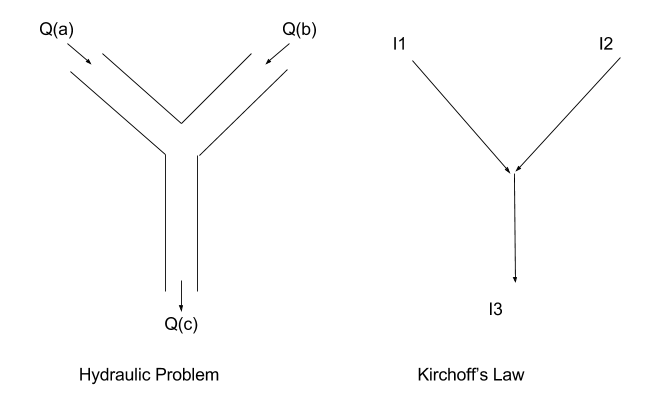Learning by example(inductive learning)

- Learning by expel is the process of learning concepts by drawing inductive inference from a set of facts. Such system defines a class for each domain with features as a facts. They are sketched into a decision tree. The system can search a decision for concept space

- Iterative Dichotomizer 3(ID3)
I) Id3 is an algorithm to generate a decision tree
II) The tree has decision nodes and leaf nodes connected by arcs.
III) It is a top-bottom approach
IV) Entropy or information gain is used to select most useful attribute for classification
H=-∑pilog2pi

- ID3 algorithm
1. Create root node
2. If all examples are +ve , create positive node and stop
3. If all exaples are –ve ,create negative node and stop
4. Otherwise
a) Calculate entropy to select root node and branch node.
b) Partition examples into subset
c) Repeat until all examples are calssified.

Example: Observing weather for 14 days: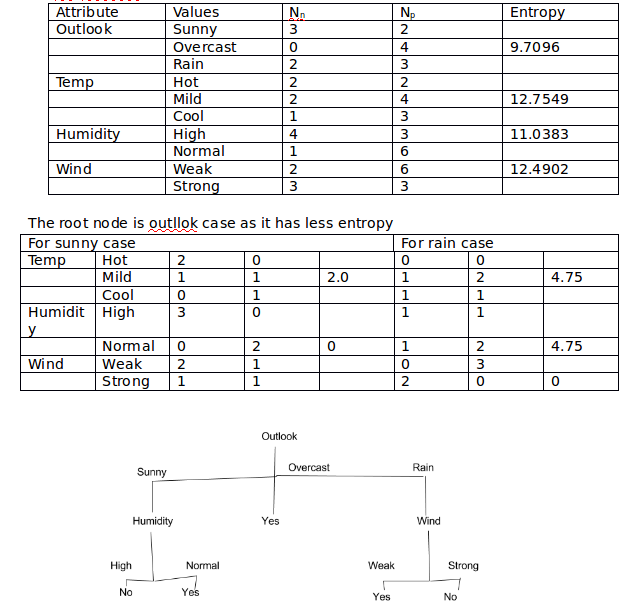Explanation based learning

- It is the approach to learn from a single example x by explaining why x is an example of the target concept
- The explanation is then generalized.

Learning Framework

The block diagram for the learning framework is shown in given figure: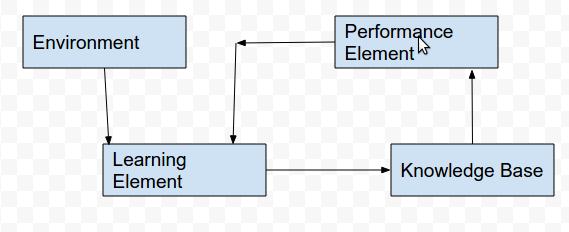Genetic Algorithm

- Genetic algorithm is based on biological evolution process; natural selection and genetic inheritance
- It generates a set of random solutions to a problem and make them complete in an area where only the fittest survive.
- Each solution in a set represent chromosome.
- A set of such solutions from a population.

Genetic Operators

1. Selection-> it replicates the most successful solutions found in a population at a rate proportional to their relative quality
2. Crossover -> it decomposes two distinct solutions and randomly mixes their part to form novel solutions
3. Mutation -> it randomly produces a candidate solutions

Algorithm

1. produce an initial population of individuals
2. evaluate the fitness of all individuals
a) select fitter individual for reproduction
b) recombine between individuals
c) mutate individuals
d) evaluate fitness of modified individuals
e) generate a new population
4. stop

Flowchart: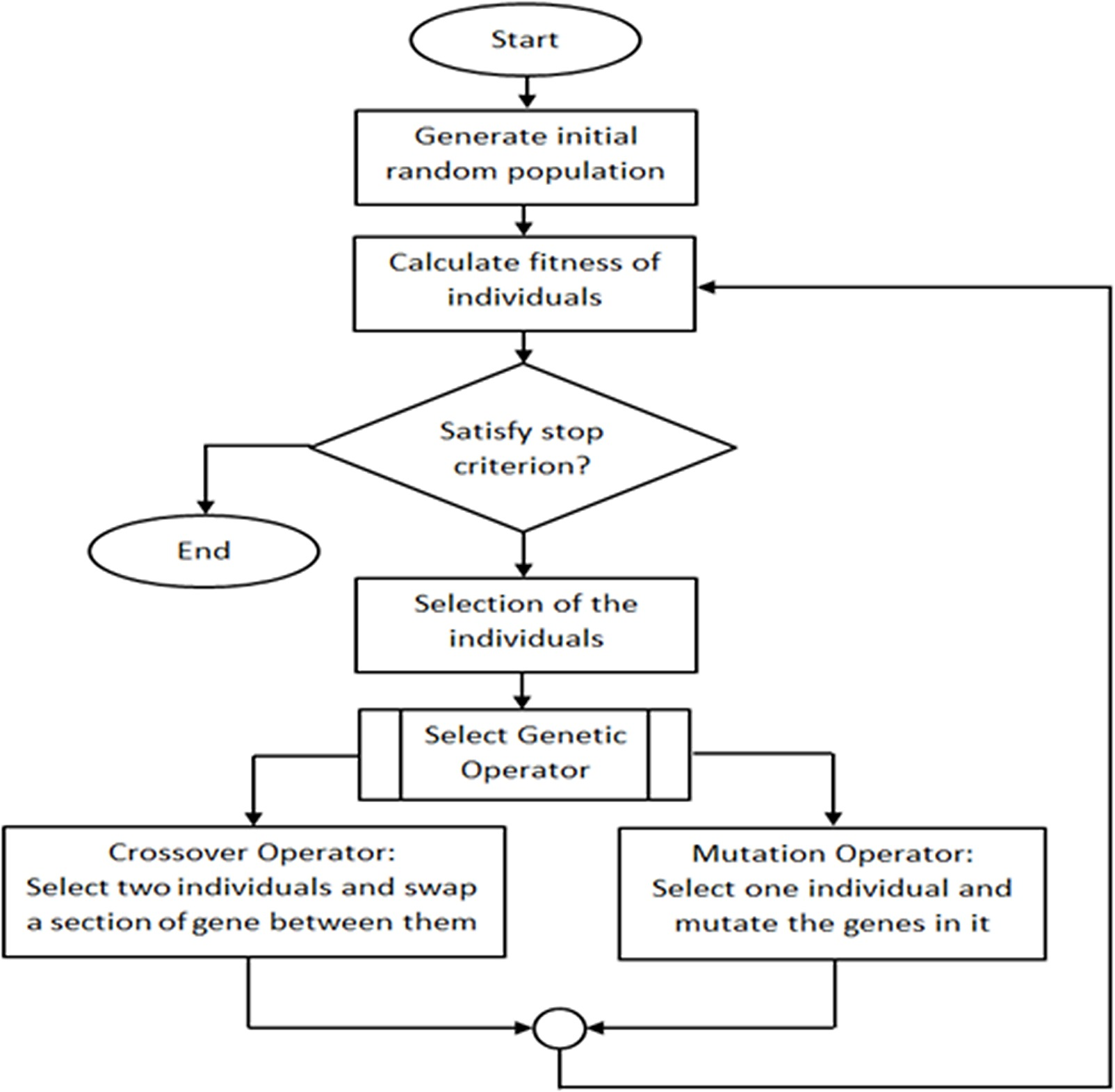Example: Generalization of input/output table

GA can be used to make machine learn and derive a function that can represent a table
Input output
A b c z
0 0 0 1
0 0 1 0
0 1 0 1
0 1 1 0
1 0 0 1
1 0 1 1
1 1 0 1
1 1 1 1

Let us introduce some weights w1,w2,and w3 and find weighted sum of inputs as:
Y=w1 a+w2 b+w3 c
Let Ze be estimated value of z such that:
Ze=0 , id y<0
Ze=1, otherwise
Assume weights can have discrete values -1,0 and +1
Now, the goal is to find values of w1,w2,and w3 that makes Ze=z all entries of a,b, and c
Let no. of correct entries in every generation be the fitness function and assume population of size 4
Solution no. w1 w2 w3 fi
1 -1 0 0 2
2 -1 0 1 4
3 -1 0 -1 4
4 1 -1 -1 5
∑ 18

On Reproduction

[{1,-1,-1}, {-1,0,1}, {-1,0,-1}, {1,-1,-1}]
f(i) = {5,4,4,5}

On Crossover (1 and 3 at site 2 ; 2 and 4 at site 1)

[{1,-1,-1}, {-1,0,-1}, {-1,-1,-1}, {1,0,1}]
f(i) = {5,4,3,6}

On Reproduction

[{1,0,1}, {1,-1,-1}, {-1,0,-1}, {1,0,1}]
f(i) = {6,5,4,6}

On crossover (1 and 2 ; 3 and 4 both at site 2)

[{1,0,-1}, {1,-1,1}, {-1,0,1}, {1,0,-1}]
f(i) = {8,5,4,8}

So, the correct solution is {1,0,-1}.

Fuzzy Learning

- Fuzzy logic is a knowledge representation technique which is used if the notions can not be defined precisely and depend upon their contexts.
- In fuzzy logic, truth value may range between completely true or completely false
- Crisp variable represent precise quantities
- Fuzzy set a of universe X is defined by function μa(x) called membership function of set a
i.e μa(x): X->[0,1]
where, μa(x)=1, if x is totally in A
=0 if x is not a
0< μa(x)<1, if x is partially in a

Fuzzy inference:

- Mamdani and Sugeno fuzzy inference
- Mamdani inference can be applied in four stages.

1. Fuzzification of i/p variable:
- The i/p variables are mapped based on their memberships to the respective fuzzy regions they belong to.

2. Rule evaluation
- The fuzzified inputs are taken and applied to the antecedents , the fuzzy operators(AND or OR) is used to obtain a single number, which is applied to consequent membership function

3. Aggregation of rule 0/p
- All the membership functions of all rule consequents previously scaled are taken and combined into a single fuzzy set for each o/p variable

4. Defuzzification
- The aggregated output fuzzy set is converted into a crisp number this is the final o/p of the system.

Example: Fuzzy room cooler
Assume that to maintain temp. of room , only the rate of flow of water needs to be controlled based on speed of fan and temp.
We define fuzzy terms for:
- Temp. as cold, cool moderate, warm, hot
- Fan speed as slack, low medium, brisk, fast
- Flow rate as strong negative , negative , low negative medium, low positive, positive and high positive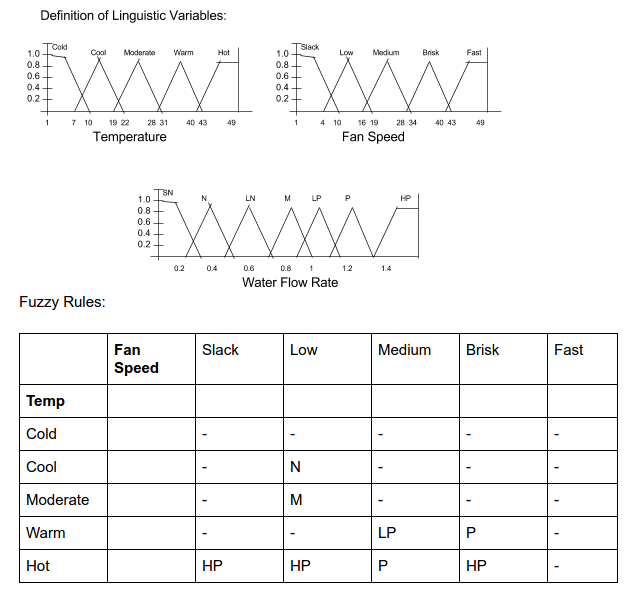Fuzzy Inference for temp 42 degree Celcius and fan speed 31 rpm:

1. Fuzzificatiion:
Parameter | Fuzzy Region | Membership
------------------------------------------------------
Temp | warm, hot | 0.142, 0.2
Fan speed | medium, brisk | 0.25, 0.286

2. Rule Evaluation:
(warm, medium)-> Lp
(warm, brisk)->p
(hot, medium)-> p
(hot, brisk)-> HP
Min(warm, medium)=0.142
Min(warm, brisk)=0.142
Min(hot, medium)=0.2
Min(hot, brisk)=0.2

3. Aggregation of Rule o/p and Defuzzification:
- The value in water flow rate profile gives an area.
- By finding the center of gravity, the crisp o/p is the desire flow rate i.e X-coordinate of centroid

Boltzmann Machines

- Boltzmann machine is a stochastic (unpredictable) recurrent neural network.
- It is stochastic extension of Hopfield network
- The network is run repeatedly by choosing a unit and setting its state after a long run at certain temp, the prob. Will depend only on states energy. This is the case of convergence

Structure of Boltzmann Machine: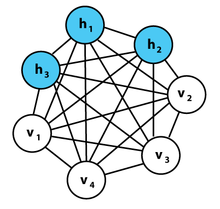Algorithm:

1) Positive phase
I) Clamp data vector on visible units
II) Lot hidden units reach thermal equilibrium
III) Sample sisj for all pairs of units
IV) Repeat for all data vectors in training set.

2) Negative phase
I) Do not champ any units
II) Let whole net. Reach thermal equilibrium
III) Sample sisj for all pairs of units
IV) Repeat many times to get good estimate

3) Weight update
I) Update each wt. by amount proportional to difference in in two phases.